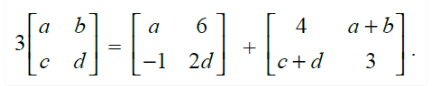# Find the value of a, b, c and d, if

Question:

Find the value of a, b, c and d, ifSolution:

Given,

$3\left[\begin{array}{ll}a & b \\ c & d\end{array}\right]=\left[\begin{array}{cc}a & 6 \\ -1 & 2 d\end{array}\right]+\left[\begin{array}{cc}4 & a+b \\ c+d & 3\end{array}\right]$

$\left[\begin{array}{ll}3 a & 3 b \\ 3 c & 3 d\end{array}\right]=\left[\begin{array}{cc}a+4 & 6+a+b \\ -1+c+d & 2 d+3\end{array}\right]$

Now,

3a = a + 4 ⇒ a = 2

3b = 6 + a + b

3b – b = 8

⇒ b = 4

And,

3d = 3 + 2d

⇒ d = 3

And,

3c = c + d – 1

2c = 3 – 1 = 2

⇒ c = 1

Hence,

a = 2, b = 4, c = 1 and d = 3